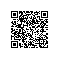# 学习C语言的数组

C语言的数组

int scores[] = {1,3};//只有两个存储空间了

int scores = { = 1, = 29};1 #include <stdio.h>
2 3 int main(int argc, const char * argv[]) {
4 5 int a;
6 printf("数组的长度：%i\n",(int)(sizeof(a)/sizeof(a)));
7 return 0;
8 }1 void printArray(int array[])
2 {
3 printf("printArray size = %lu\n", sizeof(array)); // 8 4 int length = sizeof(array)/ sizeof(int); // 2 5 printf("length = %d", length);
6 }

1.看样子，在C语言中无法将动态计算数组长度的方法模块化编程
2.在java中中的数组是可以这样的：
public class Main{
public static void main(String[] args){
int n = 10;
int a[] = new int[n];
System.out.println(a.length);
}

int a = 10;
int b[a];

C++和C语言不允许对数组的大小作动态定义。

3.但是C语言在访问数组的时候，指定索引可以用变量来指定。比如；
int a={1,2,3};
int n = 2;
printf("%d\n", a[n]);

##1.设计一个函数int arrayMax(int a[], int count)找出数组元素的最大值1 int getMax(int ages[], int length)
2 {
3 // 注意:不要假设数组以外的值位最大值,会出现意想不到的问题
4 // int max = 0;
5 // 假设数组中的第0个元素是最大  6 int max = ages;
7  8 for (int i = 0; i < length; i++) {
9 // 判断从数组中取出的值是否大于max 10 if (max < ages[i]) {
11 // 如果大于max就把当前索引对应的元素设置成最大值 12 max = ages[i];
13  }
14  }
15 16 return max;
17 }1 int getMax(int ages[], int length)
2 {
3 // 把数组中的第0个索引作为最大值  4 int max = 0;// 是一个索引  5 for (int i = 1; i < length; i++) {
6 if (ages[max] < ages[i]) {
7 max = i;
8  }
9  }
10 return ages[max];
11 }1 #include <stdio.h>
2  3 //设计一个函数int arrayMax(int a[], int count)找出数组元素的最大值
4 //面向元素具体值写算法  5 int arrayMax(int a[],int count)
6 {
7 int max = a;
8 for (int i=0; i<count; i++) {
9 max = (max>a[i])?max:a[i];
10  }
11 return max;
12 }
13 14 //面向索引写算法 15 int arrayMax2(int a[],int count)
16 {
17 //定义一个最大值的索引，比如这里的最大值索引是数组中的第一个 18 int max = 0;
19 20 for (int i=0; i<count; i++) {
21 max = (a[max]>a[i])?max:i;
22  }
23 return a[max];
24 }
25 26 int main(int argc, const char * argv[]) {
27 int a = {12,2,2,3,23};
28 printf("最大值是：%d\n",arrayMax2(a,5));
29 return 0;
30 }使用钉钉扫一扫加入圈子
+ 订阅